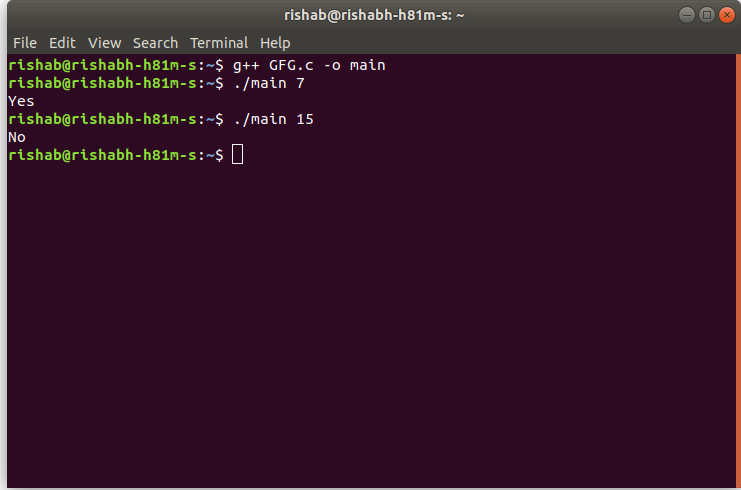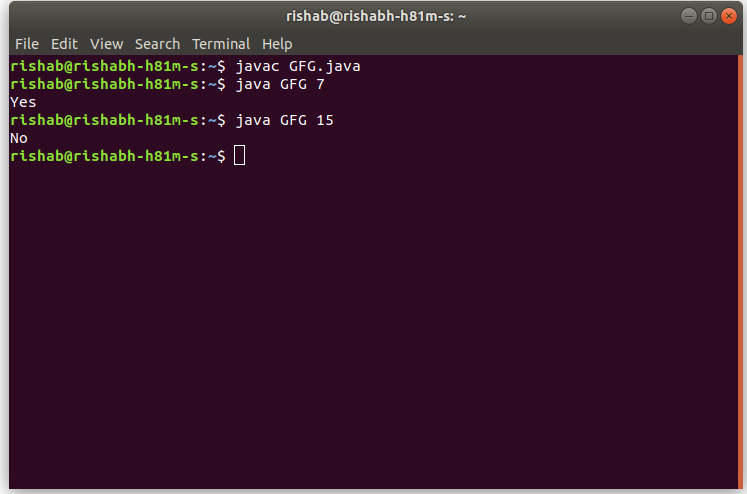Open in App
Not now

# TCS Coding Practice Question | Checking Prime Number

• Difficulty Level : Basic
• Last Updated : 16 Feb, 2023

Given a number N, the task is to check if N is a Prime Number or not using Command Line Arguments.
Examples:

```Input: N = 7
Output: Yes

Input: N = 15
Output: No```

Approach:

• Since the number is entered as Command line Argument, there is no need for a dedicated input line
• Extract the input number from the command line argument
• This extracted number will be in String type.
• Convert this number into integer type and store it in a variable, say N
• Now loop through the numbers from 2 to N/2+1, using a variable say i.
In each iteration,
• Check if i divide N completely (i.e. if it is a factor of N).
• If yes, then N is not a prime number.
• If no, then N is a prime number.
• After the loop has ended, it is found out that N is prime or not.

Note: Please note that 1 is not checked in this scenarios because 1 is neither prime nor composite.
Program:

## C

 `// C program to find ` `// the Prime Numbers from 1 to N ` `// using command line arguments ` ` `  `#include ` ` `  `#include /* atoi */ ` ` `  `// Function to check if x is prime ` `int` `isPrime(``int` `x) ` `{ ` `    ``int` `i; ` ` `  `    ``// Loop to check if x has any factor ` `    ``// other than 1 and x itself ` `    ``for` `(i = 2; i < x / 2 + 1; i++) { ` `        ``if` `(x % i == 0) { ` `            ``// Since i is a factor of x ` `            ``// x is not prime ` `            ``return` `0; ` `        ``} ` `    ``} ` ` `  `    ``// x is prime ` `    ``return` `1; ` `} ` ` `  `// Driver code ` `int` `main(``int` `argc, ``char``* argv[]) ` `{ ` ` `  `    ``int` `n; ` ` `  `    ``// Check if the length of args array is 1 ` `    ``if` `(argc == 1) ` `        ``printf``(``"No command line arguments found.\n"``); ` `    ``else` `{ ` ` `  `        ``// Get the command line argument and ` `        ``// Convert it from string type to integer type ` `        ``// using function "atoi( argument)" ` `        ``n = ``atoi``(argv); ` ` `  `        ``// Check if n is prime ` `        ``if` `(isPrime(n) == 1) ` `            ``printf``(``"Yes\n"``); ` `        ``else` `            ``printf``(``"No\n"``); ` `    ``} ` ` `  `    ``return` `0; ` `} `

## Java

 `// Java program to reverse a string ` `// using command line arguments ` ` `  `class` `GFG { ` ` `  `    ``// Function to check if x is prime ` `    ``public` `static` `int` `isPrime(``int` `x) ` `    ``{ ` `        ``int` `i; ` ` `  `        ``// Loop to check if x has any factor ` `        ``// other than 1 and x itself ` `        ``for` `(i = ``2``; i < x / ``2` `+ ``1``; i++) { ` `            ``if` `(x % i == ``0``) { ` `                ``// Since i is a factor of x ` `                ``// x is not prime ` `                ``return` `0``; ` `            ``} ` `        ``} ` ` `  `        ``// x is prime ` `        ``return` `1``; ` `    ``} ` ` `  `    ``// Driver code ` `    ``public` `static` `void` `main(String[] args) ` `    ``{ ` ` `  `        ``// Check if length of args array is ` `        ``// greater than 0 ` `        ``if` `(args.length > ``0``) { ` ` `  `            ``// Get the command line argument and ` `            ``// Convert it from string type to integer type ` `            ``int` `n = Integer.parseInt(args[``0``]); ` ` `  `            ``// Check if n is prime ` `            ``if` `(isPrime(n) == ``1``) ` `                ``System.out.println(``"Yes"``); ` `            ``else` `                ``System.out.println(``"No"``); ` `        ``} ` `        ``else` `            ``System.out.println(``"No command line "` `                               ``+ ``"arguments found."``); ` `    ``} ` `} `

Output:

• In C:• In Java:Time Complexity: O(N)
Auxiliary Space: O(1)

My Personal Notes arrow_drop_up
Related Articles